New Near Open Set In Topological Space | OMICS International
Journal of Physical Mathematics
All submissions of the EM system will be redirected to Online Manuscript Submission System. Authors are requested to submit articles directly to Online Manuscript Submission System of respective journal.

# New Near Open Set In Topological Space

Sayed MEL* and Mansour FHAL

Department Mathematics, College of Science and Arts, Najran University, Saudi Abrabia

*Corresponding Author:
Sayed MEL
Professor, Department Mathematics
College of Science and Arts
Najran University, Saudi Abrabia
Tel: +966 17 542 8888
E-mail: [email protected]

Received Date: November 07, 2016; Accepted Date: November 28, 2016; Published Date: November 30, 2016

Citation: Sayed MEL, Mansour FHAL (2016) New Near Open Set In Topological Space. J Phys Math 7:204. doi: 10.4172/2090-0902.1000204

Copyright: © 2016 Sayed MEL, et al. This is an open-access article distributed under the terms of the Creative Commons Attribution License, which permits unrestricted use, distribution, and reproduction in any medium, provided the original author and source are credited.

Visit for more related articles at Journal of Physical Mathematics

#### Abstract

The aim of this paper is to introduce new class of near open sets namely, b*-open set. And studs same of their properties, also we study the relation between this class among this classes. Also, we introduce some topological properties and we shall study same of their properties.

#### Keywords

b*-open set, b*-interior, b*-closure, b*-boundary, b*- neighbourhood.

#### Introduction

Topological ideas are present not only in almost all areas of today mathematics for example biochemistry  information systems  and others for more fields of topology applications see ref.  and its related links. The subject of topology itself consist of several different branches such as point set topology, algebraic topology and differential topology which have relatively little in common this richness of applications and difference between branches of topology. implied a difficulty to give an accurate definition for topology. In 1937  M.H. Stone introduced the concept of regular open sets. In 1963  Levine introduced the concept of semi open sets. In 1965  Najasted introduced the concept of α-open sets. In 1982 [7,8] Mashhour, Abd El-Monsef and El-Deeb introduced the concept of pre-open sets. In 1983  Abd El-Monsef and et al. introduced the concept of β-open sets. In 1996  Andrijevic introduced the concept of b-open sets, In 2013  Hariwan Z Ibrahim introduced the concept of Bc-Open Set.

Definition 1.1: A subset A of topological space(X,τ) is called:A⊆int(cl(int(A)))

(1) α-open if A⊆int(cl(int(A))) 

(2) preopen if A⊆int(cl(A)) 

(3) semi open if A⊆cl(int(A)) 

(4) Regular open if A=int(cl(A)) β 

(5) β-open (or semi pre open) if, A⊆ (cl(int(cl(A))) [9-15]

(6) b-open. A⊆cl(int(A) ) Ç int(cl(A) ) 

(7) A subset A of a space X is called Bc-open if for each x ∈ A ∈bO(X), there exists a closed set F such that x ∈ F ⊂ A 

Remark 1.1: The complement of a α-open (resp. preopen, semi open, Regular open, β-open and b-open) sets is called α-closed (resp. pre closed, semi closed, Regular closed, β-closed and b-closed ) sets. The intersection of all α-closed (resp. pre closed, semi closed, Regular closed, β-closed and b-closed ) sets containing A is called the α-closure (resp. pre-closure, semi-closure, Regular closure, β-closure and b-closure ) of A and is denoted by αcl(A) (resp. pcl (A), scl(A), Rcl(A), βcl(A) or spcl (A), and bcl(A)).

The union of all a α-open (resp. preopen, semi open, Regular open, β-open, and b-open ) sets contained in A is called α-intrior (resp. pre-intrior, semi-intrior, Regular intrior, β-intrior and b-intrior ) of A and is denoted by αint(A) (resp. pint (A), sint (A), Rint(A), βint(A) or spint (A), and bint (A)). The family of all a α-open (resp. α-closed, preopen, pre closed, semi open, semi closed, Regular open, Regular closed, β-open, β-closed and b-open, b-closed) sets is denoted by αO(X) (resp αC(X), PO(X), PC(X), SO(X), SC(X), RO(A),C(A), βO(A), βC(A), bO(A) and bC(A)).

Proposition 1.1: For sub set A,B a spase(X,τ), the following statmant hold :

(1) pcl(A) = A∪cl(int(A)), pint(A) = A∩int(cl(A)) .

(2) spcl(A) = A∪int(cl(int(A))), spint(A) = A∩cl(int(cl(A))) .

(3) pcl(A∪ B) ⊆ pcl(A)∪ pcl(B) , spcl(A∪ B) ⊆ spcl(A)∪ spcl(B) [12,13].

(4) pint(A∩ B) ⊆ pint(A)∩ pint(B), pint(A∪ B) ⊇ pint(A)∪ pint(B) .

(5) X / (int(A)) = cl(X / (A)), int(X / A) = X / cl(A) .

2 b*-Open sets

Definition 2.1: let (X,τ) be topological space. Then a subset A of Xis said to be

1. a b*-Open set if A ⊆ cl(int(cl(A)))∪int(cl(A)).

2. a b*-closed set if A ⊇ int(cl(int(A)))∩cl(inl(A)).

The family of all b*-Open set (resp. b*-closed set)subsets of a spase (X,τ) will be as always denoted by bO*(X) (resp. bC*(X))

Example 2.1: Let X={a,b,c,d}with topology τ={X,ϕ,{a},{a,b},{a,c,d}}. Then the classes of b*-open set and b*-closed set

b*O(X ) = {X ,Φ,{a},{a,b},{a,c},{a,d},{a,d},{a,b,c},{a,b,d},{a,c,d}},

and

b*C(X ) = {X ,Φ,{b},{c},{d},{c,d},{b,d},{b,c},{b,c,d}}

Proposition 2.1: Let A be a sub set of a space (X,τ). Then (1) Every preopen (resp. Bc-open) set is b*-open

Remark 2.1: The converse of the above propsition is not necessarily true as shown by the following example.

Example 2.2: Let X={a,b,c} with topology τ={X,Φ {a},{b},{a,b}}. Then

(1) A subset {a,c} of X is b*-open but not preopen.

(2) A subset {a} of X is b*-open but not Bc-open.

Remark 2.2: According to Definition (2.1) and Proposition (2.1), the following diagram holds for a subset A of a space X :

Lemma 2.1: Let (X,τ) be topological space. Then the following statements are hold

(1) The union of b*-Open sets is b*-open

(2) The intersection of b*-closed sets is b*-closed

Proof: (1) let {Ai,i∈I} be a family of b*-Open sets. Then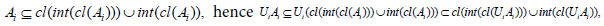for all iI. Thus UiAi is b*-Open

(2) let {Ai,i∈I} be a family of b*-Closed sets. Then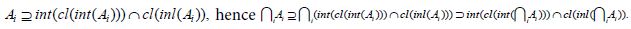for all i∈I. Thus ∩ Ai is b*closed

Remark 2.3: The intersection of any two b*-open sets is not b*-open. Let X={a,b,c,d},

τ={X,Φ {a},{c,d},{a,c,d}}. Then A={a,b} and B={b, c} are b*-open sets, but A ∩ B={b} is not b*-open.

Definition 2.2: Let (X,τ) be topological space. Then:

(1) The union of all b*-open sets of X contained in A is called the b*-interior of A and is denoted by b*-int(A).

(2) The intersection of all b*-closed sets of X contained in A is called the b*-closure of A and is denoted by b*-Cl(A).

Example 2.3: Let X={a,b,c,d} with topology τ={X,Φ {a},{c},{a,c}}. and A={a,b}, B={a, c} are b*open then

b*− int(A) = {a,b},b* − int(B) = {a,c} and b* − cl(A) = {a,b},b* − cl(B) = X

Theorem 2.1: Let (X,τ) be topological space and A ⊂ X, then the following statement are equivalent:

(1) A is a b*-open set,

(2) A=spint(A)∪ pint (A)

Proof: (1)→(2). Let Α be a b*-open set. Then A ⊆ cl(int(cl(A)))∪int(cl(A)) , hence by proposition (1.1).

spint(A)∪ pint(A) = (A∩cl(int(cl(A)))∪(A∩int(cl(A))) = A∩ (cl(int(cl(A)))∪int(cl(A))) = A

(2)→(1). Suppose that A=spint(A)∪ pint (A). Than by proposition (1.1)

A = (A∩cl(int(cl(A)))∪(A∩int(cl(A))) ⊂ cl(int(cl(A)))∪int(cl(A)) . Therefore, Α is a b*-open.

Theorem 2.2: Let (X,τ) be topological space and A ⊂ X, then the following statement are equivalent:

(1) Α is a b*-closed set,

(2) A=spcl(A)∩pcl(A)

Proof: (1)→(2) Let A be a b*-closed set. Then A ⊇ int(cl(int(A)))∩cl(int(A)) , hence by proposition(1.1).

spint(A)∩ pint(A) = (A∪cl(int(cl(A)))∩(A∪int(cl(A))) = A∪ (cl(int(cl(A)))∩int(cl(A))) = A

(2)→(1). Suppose that A=spcl(A)∩pcl(A). Than by proposition (1.1)

A = (A∪int(cl(int(A)))∩(A∪cl(int(A))) ⊃ int(cl(int(A)))∩cl(int(A)) . Therefore, A is a b*-closed.

Theorem 2.3: Let A be a supset of a space (X,τ). Then

(1) b* − cl(A) = spcl(A)∩ pcl(A) ,

(2) b* − int(A) = spint(A)∪ pint(A) .

Proof: (1) It is easy to see that b* − cl(A) ⊆ spcl(A)∩ pcl(A) . Also spcl(A)∩ pcl(A) = (A∪int(cl(int(A)))∩(A∪cl(int(A)))= A∪ (int(cl(int(A)))∩cl(int(A))). But, b*- cl(A) is b*-closed, hence x A∪(int(cl(int(A)))∩cl(int(A))) ⊂ A∪b* − cl(A) = b* − cl(A) = b* Thus A∪(int(cl(int(A)))∩cl(int(A))) ⊂ A∪b* − cl(A) = b* − cl(A) = b* there for, spcl(A)∩ pcl(A) ⊂ b* − cl(A) . So b* − int(A) ⊆ spint(A)∪ pint(A) .

(2) It is easy to see that b* − int(A) ⊆ spint(A)∪ pint(A) . Also spint(A)∪ pint(A) = (A∩cl(int(cl(A)))∪(A∩int(cl(A))) = A∩ (cl(int(cl(A)))∪int(cl(A))). But, b*-int(A) is b*-open, hence b* − int(A) ⊂ cl(int(cl(b* − int(A))))∪int(cl(b* − int(A))) ⊂ (cl(int(cl(A)))∪int(cl(A))) . Thus A∩(cl(int(cl(A)))∪int(cl(A))) ⊂ A∩b* − int(A) = b* − int(A) there for spint(A)∪ pint(A) ⊂ b* − int(A) .

So b − int(A) = spint(A)∪ pint(A) .

Theorem 2.4: Let A be a supset of a space (X,τ). Then

(1) A is a b*-open set if and only if A=b*-int(A)

(2) A is a b*-closed set if and only if A=b*-cl (A)

Proof: (1) Let A be a b*-open set. Then by theorem (2.1), A=spint(A)∪ ∪ pint (A) and by theorem (2.3), we have A=b*-int(A) Conversely, let A=b*-int(A) Then by theorem(2.3), A=spint(A)∪ pint (A) and by theorem (2.1), A is b*-open A=spint(A)∪ pint (A)

(2) Let A be a b*-closed set. Then by theorem (2.1), A=spcl(A)∩pcl(A)and by theorem (2.3), we have A=b*-cl (A) Conversely, let A=b*-cl (A) Then by theorem (2.3), A=spcl(A)∩pcl(A) and by theorem (2.1), A is b*-closed

Theorem 2.5: Let A and B be a subsets of a space(X,τ). Then the following are hold

(1) b* − cl(X \ A) = X \ b* − int(A).

(2) b* − int(X \ A) = X \ b* − cl(A) .

(3) If A ⊆ B, then x∈b*-cl (A)

(4) x∈b*-cl (A)if and only if there exists a b*-open set U and x∈U such that U ∩ A ≠ϕ.

(5) x∈b*-int(A) if and only if there exists a b*-open set G and x∈G such that x∈G⊆A

(6) b* − cl(b* − cl(A)) = b* − cl(A) and b* − int(b* − int(A)) = b* − int(A).

(7) b* − cl(A)∪b* − cl(B) ⊆ b* − cl(A∪ B) and b* − int(A)∪b* − int(B) ⊆ b* − int(A∪ B)

(8) b* − int(A∩ B) ⊆ b* − int(A)∩b* − int(B) , b* − cl(A∩ B) ⊆ b* − cl(A)∩b* − cl(B)

Proof: (1) Since (X\A) ⊆ X, by theorem (2.4) b* − cl(X \ A) = spcl(X \ A)∩ pcl(X \ A) and by proposition (1.1) b* − cl(X \ A) = (X \ spint(A))∩(X \ pint(A)) = X \ (spint(A)∪ pint(A)) , hence by theorem (2.4), b* − cl(X \ A) = X \ b* − int(A)

(2) Since (X\A) ⊆ X, by theorem (2.4) b* − int(X \ A) = spint(X \ A)∪ pint(X \ A) and by proposition (1.1) b* − int(X \ A) = (X \ spcl(A))∪(X \ pcl(A)) = X \ (spcl(A)∩ pcl(A)) , hence by theorem (2.4), b* − int(X \ A) = X \ b* − cl(A)

(3) Since, b* − cl(A) = spcl(A)∩ pcl(A) and A ⊆ B, b* − cl(A) = spcl(A)∩ pcl(A) ⊆ spcl(B)∩ pcl(B) = b* − cl(B)

(4) Let x∉b*-cl(A)then x∉∩F where F is b*-closed with A ⊂ F, so x ∉ X\∩F and X\∩F is a b*-open set containing x and hence (X \∩F)∩ A ⊆ (X \∩F)∩(∩F) =φ . Converly, suppose that exists a b*-open set containing x with A ∩ U=ϕ.

Then A⊆X/U and X/U is a b*-closed. Hence x∉b*-cl(A).

(5) Necessity. Let x∈ b*-int(A). Then x∈∪{G :G is b* − open G ⊆ A} and hence there exists b*-open set G such that x∈G⊆A sufficiency. Let G be a b*-open set such that x∈G⊆A. Then A=∪{G: x∈G} which is the union of b*-open set. There for, x∉b*-cl(A).

(6) Since b* − cl(b* − cl(A)) = spcl(b* − cl(A))∩ pcl(b* − cl(A)) . by theorem (2.4).

spcl(spcl(A)∩ pcl(A))∩ pcl(spcl(A)∩ pcl(A)) ⊆ (spcl(A)∩spcl( pcl(A)))∩ pcl(spcl(A)∩ pcl(A)) = spcl(A)∩ pcl(A) = b* − cl(A) hence: b* − cl(b* − cl(A)) ⊆ b* − cl(A) . But, b* − cl(A) ⊆ b* − cl(b* − cl(A)) , there for, b* − cl(b* − cl(A)) = b* − cl(A)

(7) Since A⊆ A∪B and B⊆ A∪B we have b*-cl (A) ⊆b*-cl(A∪B) and b*-cl (B) ⊆b*-cl(A∪B). There for b*-cl (A) ∪ b*-cl (B)⊆ b*-cl(A∪B) A ⊆(A∪B) and and B⊆(A∪B) we have b*-int(A) ⊆b*-int(A∪B) and b*-int(B) ⊆b*-int(A∪B). There for b*-int(A) ∪ b*-int(B) ⊆b*-int(A∪B).

(8) Since A ⊇ A∩B and B ⊇ A∩B we have b*-cl (A) ⊇b*-cl(A∩B) and b*-cl (B) ⊇ b*-cl(A∩B), b*-cl (B) ⊇ b*- cl ((A∩B). There for for b*-cl (A) ∩ b*-cl (B) b*-cl(A∩B) and A (A∩B) and B⊇(A∩B) we have b*-int(A) ⊇b*-int(A∩B) and b*-int(B) ⊇ b*-int(A∩B).There for b*-int(A)∩b*-int(B) ⊇b*- int(A∩B).

Remark 2.4: The inclusion relation in part (6),(7) of the above theorem cannot be replaced by equality as shown by the following example.

Example 2.4: Let X={a,b,c,d} with topologyτ={X,Φ {a},{b},{c}{a,b},{a,c}{b,c}{b,d}{a,b,c}{a,b,d}{b,c,d}}.

Then (A∪B)={a,b,d}

(1) If A={a,b},B={d} and (A∪B)={a,b,d}, then b*-int(A)=A b*-int(B)=ϕ and b*-int(A∪B)={a,b,c} So, b*-int(A∪B) b*-int(A) ∪ b*-int(B)

(2) If C={b}, B={d} and (B∩C)=ϕ, then b*-cl (C)={b,d} b*-cl (B)=B and b*-cl(B∩C)=ϕ, there for, b*-cl (B) ∩ b*-cl (C) b*-cl(B∩C)

Example 2.5: Let X={a,b,c,d} with topology τ={X,Φ {a},{b,c},{a,b,c}} then

(1) If A={a,b}, B={a,c} and(A∪B)={a,b,c}, then b*-cl (A)=A b*-cl (B)=B and b*-cl(A∪ B)=X So, b*-cl(A∪B) b*-cl(A) ∪ b*-cl(B)

(2) If C={a,d}, D={b,d} and (B∩C)={d}, then b*-int(C)=C b*-int(D)=D and b*-int(C∩D)=ϕ b*-int(C) ∪ b*-int (D) b*-int(C∩D) 3 Some Topological Operations.

Definition 3.1: Let (X,τ) be a space and A ⊂ X. Then the b*-boundary of A (briefly, b*-b(A)) is given by b*-b(A))=b*-cl (A)) ∩ b*-cl(X/A)

Example 3.1: From Example (2.1) we have A={a} B={a,b} C={a,b,d} then b*-b(A))={b,c,d}, b*-b(B))={c,d}and b*-b(C))={c}

Remark 3.1: For any subset A of a space (X,τ) we have b*-b(A)⊆ b(A) and b*-b(A)⊆ p-b(A).

The inclusion of the above remark can be replaced as shows in the following example.

Example 3.2: From Example (2.3) and A={a,b} then b*-b(A)=ϕ, p-b(A)={b,d} we have p-b(A)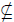b*-b(A).

Theorem 3.1: If A is a sub sets of a space (X,τ), then the following statement are hold:

(1) b* − b(A)) = b* − b(X \ A)).

(2) b* − b(A)) = b* − cl(A)) \ b* − int(A).

(3) b* − b(A))∩b* − int(A) = Φ.

(4) b* − b(A))∪b* − int(A) = b* − cl(A).

Proof: (1) Since b* − b(A)) = b* − cl(A))∩b* − cl(X \ A) = b* − b(X \ A)) = b* − cl(X \ A))∩b* − cl(A)

(2)Since, b* − b(A)) = b* − cl(A))∩b* − cl(X \ A) = b* − cl(A))∩(X \ b* − intl(A)) = (b* − cl(A)∩ X ) \ (b* − cl(A)∩ X ) \ (b* − cl(A)∩b* − intl(A)) = b* − cl(A) \ b* − int(A).

(3) Also, by using(2) b* − b(A))∩b* − int(A) = (b* − cl(A) \ b* − int(A))∩b* − int(A) = (b* − cl(A)∩b* − int(A)) \ b* − int(A) = b* − int(A) \ b* − int(A) = Φ.

(4) By using(3) b* − b(A))∪b* − int(A) = (b* − cl(A) \ b* − int(A))∪b* − int(A) = b* − cl(A).

Theorem 3.2: If A is a sub sets of a space (X,τ), then the following

statement are holds:

(1) A is a b*-open set if and only if A∩b*-b(A)=Φ

(2) A is a b*-closed set if and only if b*-b(A) ⊂A

(3) A is a b*-clopen set if and only if b*-b(A)=Φ.

Proof: (1) let A is a b*-open set. Then A=b*- int(A) hence by theorem (3.1)

A∩b*-b(A)=b*-int(A)∩b*-b(A)=Φ Conversely, let A∩b*-b(A)=Φ then by theorem (3.1),

A∩(b* − cl(A) \ b* − int(A)) = (A∩(b* − cl(A)) \ (A∩b* − int(A)) = A / b* − int(A) = Φ. so, A=b*-int(A) and hence A is b*-open.

(2) let A is a b*-closed set. Then A=b*-cl(A), by thearom (3.1), but b* − b(A) = (b* − cl(A) \ b* − int(A)) = A \ b* − int(A), then b*-b(A) ⊂A Conversely

let b*-b(A) ⊂A. Then by theorem(3.1), b* − cl(A) = b* − b(A))∪b* − int(A) ⊂ A∪b* − int(A) = A thus b*-cl(A) ⊂A and A⊂ b*-cl(A) there for, A=b*- cl(A),

(3) let A is a b*-clopen set. Then A=b*-int(A), and A=b*-cl(A), hence by thearom (3.1), b* − b(A) = (b* − cl(A) \ b* − int(A)) = A \ A = Φ Conversely, suppose that b*-b(A)=Φ.Then b* − b(A) = (b* − cl(A) \ b* − int(A)) = Φ , and hence, A is a b*-clopen set.

Definition 3.2: Let (X,τ) be a space and A ⊂ X.Then the setX\(b*-cl(A)) is called the b*-exterior of A and is denoted by b*-ext(A). Each point p∈ X is called an b*-exterior point of A, if it is a b*-interior point of X\A.

Example 3.3: let X={a,b,c,d} with topology τ={X,Φ {a},{b},{a,b},{ b,c,d}}

If A={a} B={a,c} C={ b,c,d} then we have

b*-ext(A)={ b,c,d}, b*-ext(B))={ b, d} and b*-b(C))={a}

Remark 3.2: For any topology space (X,τ) and A ⊂ X, we have ext(A)⊆p-ext(A)⊆b*-ext(A)

Proof: Since b*-cl (A) ⊆ cl(A), then X \ cl(A) ⊆ X \ b* − cl(A) and int(X \ A ⊆ b* − int(X \ A) i.e ext(A) ⊆ b* − ext(A) . Since ext(A) ⊆ p − ext(A) , then we have p − ext(A) ⊆ b* − ext(A) . This implies that the relation hold.

Example 3.4: Let X={a,b,c,d} with topology τ={X,Φ {c},{d}{c,d},{b,c}{b,c,d}}. And A={b,d}, B={c} we have b* − ext(A) = {a,c}, p − ext(A) = {c}, p − ext(B) = {b,d},ext(B) = {d}

Theorem 3.3: If A and B is two sub sets of aspace (X,τ), then the following statements are hold: ext(A) ∪ b*

(1) b*-ext(A)=b*-int(A).

(2) b*-ext(A) is b*-open

(3) b*-ext(A)∪ b*-int(A)=.

(4) b*-ext(A)∩ b*-b(A)=.

(5) b*-ext(A)∪ b*-b*-b(A)=b* cl(X \ A) .

(6) {b* − int(A),b* − b(A)and b* − ext(A)} from apartition of X.

(7) If A ⊆ B, then b* − ext(B) ⊆ b* − ext(A)

(8) b* − ext(A∪ B) ⊆ b* − ext(A)∪b* − ext(B).

(9) b* − ext(A∩ B) ⊇ b* − ext(A)∩b* − ext(B).

(10) b* − ext(X ) = Φand b* − ext(φ ) = X.

Proof: (1) by Definition (3.2) b* − ext(A) = X \ b* − cl(A) = b* − int(X \ A) .

(2) From (1) b* − ext(A) = b* − int(X \ A) . Since b*-int(A) is the union of all b*-open sets of X contained in A thus b*-ext(A) is b*-open

(3) Since b* − ext(A)∩b* − int(A) = X \ b* − cl(A)∩b* − int(A) = b* − int(X \ A)∩b* − int(A) =φ

(4) By theorem (3.1), b* − ext(A)∩b* − b(A) = b* − int(X \ A)∩b* − b(X \ A) = Φ.

(5) Also, by theorem (3.1)

b* − ext(A)∪b* − b(A) = b* − int(X \ A)∪b* − b(X \ A) = b* − cl(X \ A).

(6) From (3),(4) we have b* − ext(A)∩b* − int(A) = Φ and b* − ext(A)∩b* − b(A) = Φ . Then by theorem (3.1) then b* − b(A))∩b* − int(A) = Φ. Now, we need to prove that b* − int(A)∪b* − b(A)∪b* − ext(A) = X hence from(5) b* − ext(A)∪b* − b(A) = b* − cl(X \ A) then b* − int(A)∪b* − cl(X \ A) = b* − int(A)∪ X \ b* − int(A) = X.

(7) let A ⊆ B then (b* − cl(A)) ⊆ (b* − cl(B)) and hence

X \ (b* − cl(B)) ⊆ X \ (b* − cl(A)) . So b* − ext(B) ⊆ b* − ext(A) .

(8) b* − ext(A∪ B) = X \ (b* − cl(A∪ B)) ⊆ X \ (b* − cl(A)∪(b* − cl(B)) == (X \ (b* − cl(A)))∩(X \ (b* − cl(B))) = b* − ext(A)∩b* − ext(B) ⊆ b* − ext(A)∪b* − ext(B).

(9) b* − ext(A∩ B) = X \ (b* − cl(A∩ B)) ⊃ X \ (b* − cl(A)∩(b* − cl(B)) = (X \ (b* − cl(A)))∪(X \ (b* − cl(B))) = b* − ext(A)∪b* − ext(B) ⊇ b* − ext(A)∩b* − ext(B).

(10) b* − ext(X ) = X \ (b* − cl(X )) = X \ X =φ and b* − ext(φ ) = X \ (b* − cl(φ )) = X \φ = X

Remark 3.3: The inclusion relation in part (5),(6) of the above theorem cannot by replaced by equality as is show by the following example.

Example 3.5: From Example (2.1) we have A={b,c}andB={a,c} then b*-ext(A)={a,b},b*-ext(B)=Φ but b* − ext(A∪ B) = Φ. Therefor, b* − ext(A)∪b* − ext(B)b* − ext(A∪ B). Also. b* − ext(A∩ B) = {a,b, d} , hence b* − ext(A∩ B)b* − ext(A)∩b* − ext(B).

Definition 3.3: If A is a subset of a space (X,τ), then a point pX is called a b*-limit point of a set A ⊂ X if every b*-open set G∈X containing p contains a point of A other than p. The set of all b*- limit point of A is called an b*-derived set of A and is denoted by b*-d(A)

Example 3.6: let X={a,b,c,d} with topology τ={X,Φ {a},{c,d},{a,c,d}} and If A={a,d} B={a,c,d} the b*-d(A))={Φ}, and b*-d(B))={b}.

Theorem 3.4: If A and B is two sub sets of aspace (X,τ), then the following statements are hold:

(1) IfA ⊂ B,then b* − d(A) ⊂ b* − d(B).

(2) A is a b*-closed set if and only if it cantains each of its b*-limit point.

(3) b* − cl(A) = A∪b* − d(A).

(4) b* − d(A∪ B) ⊃ b* − d(A)∪b* − d(B)

(5) b* − d(A∩ B) ⊂ b* − d(A)∩b* − d(B)

Proof: (1) By definition (3.3), we have p∈ b*-d(A) if and only if G ∩ (A\{P}) ≠ ϕ, for every b*-open set G containing p. But A ⊂ B, then G ∩ (B\ {P}) ≠ ϕ, for every b*-open set G containing p. Hence, so p∈ b*-d(B)

There for b*-d(A)⊂ b*-d(B)

(2) Let A be b*-closed set and p∉A then p∈(X/A) which is b*-open, hence there exists b*-open(X\A) such that

(X/A)∩A=ϕ so p∉ b*-d(A), there for b*-d(A)⊂A. Conversely, suppose that b*-d(A)⊂A and p∉A. Then p∉ b*-d(A), hence there exists b*-open set G containing p such that G ∩ A=ϕ and hence

X \ A =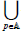{G,G is b* open there for A is b*-closed

(3) Since, b* − d(A) ⊂ b* − cl(A) and A ⊂ b* − cl(A) b* − d(A)∪ A ⊂ b* − cl(A) .

Conversely, suppose that p∉ b*-d(A)∪A Then p∉ b*-d(A), p∉A and hence there exists b*-open set G containing p such that G ∩ A=ϕ. Thus p∉ b*-cl(A) which implies thet b* − cl(A) ⊂ b* − d(A)∪ A , there for, b* − cl(A) = b* − d(A)∪ A.

(5) Since A⊇A∩B and B⊇A∩B we have b*-d(A)⊇b*-d(A∩B) and b*-d(B)⊇b*-d(A∩B). There for b*-d(A)∩b*-d(B)⊇b*-d(A∩B).

Definition 3.4: Let (X,) be a space and A⊆X. Then the b*-border of A (briefly, b*-Bd(A)) is given by b*-Bd(A))=A\b*-int(A).

Example 3.7: Let X={a,b,c,d} with topologies τ={X,,{d},{a,d},{c,d},{b,d},{b,c,d},{a,b,d},{a,c,d}}. If A={a,c}, B={c,d} and C={b,c}, then b*- Bd(A)={a,c}, b*-Bd(B)=ϕ and b*-Bd(c)={c}.

Theorem 3.5: For a subset A of a space and X, the following statements are hold:

(1) A = b* − int(A)∪b* − Bd(A)) ,

(2) b* − int(A)∩b* − Bd(A)) =φ ,

(3) b* − Bd(X )) = b* − Bd(φ )) =φ,

(4) b* − Bd(b* − int(A)) =φ ,

(5) b* − int(b* − Bd(A)) =φ ,

(6) b* − Bd(b* − Bd(A)) = b* − Bd(A)) ,

(7) b* − Bd(A)) = A∩b* − cl(X \ A) ,

(8) b* − Bd(A)) = b* − d(X \ A) ,

Proof: (1)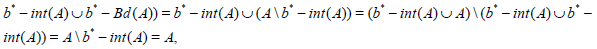(2)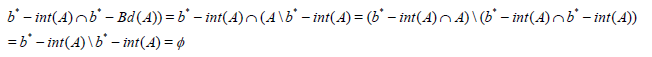(3) b* − Bd(X )) − X \ b* − int(X ) = X \ X =φ and b* − Bd(φ )) =φ \ b* − int(φ ) =φ \φ =φ.

(4) b* − Bd(b* − int(A)) = b* − int(A) \ b* − int(A) =φ .

(5)Since, b* − int(b* − Bd(A)) = b* − int(A \ b* − int(A)) = b* − int(A) \ b* − int(b* − int(A)) = b* − int(A) \ b* − int(A) =φ

(6) Since, b* − Bd(b* − Bd(A)) = b* − Bd(A) \ b* − int(b* − Bd(A)) = b* − Bd(A) \φ = b* − Bd(A)

(7) Also, from Theorem (2.5), b* − Bd(A)) = A \ b* − int(A)) = A \ (X \ b* − cl(A)) = A∩b* − cl(X \ A) .

(8) Further, from Theorem 2.3.1 b* − Bd(A)) = A \ b* − int(A)) = A \ (A \ b* − d(A)) = b* − d(X \ A) .

Theorem 3.6: For a subset A of a space and X, the following statements are equivalent

(1) A is b*-open,

(2) A=b*-int(A),

(3) b*-Bd(A)=ϕ.

Proof: (1)→(2)Obvious from Theorem (2.4).

(2)→(3). Suppose that A=b*-int(A). Then by Definition (3.4),

b*-Bd(A)=b*-int(A)\b*-int(A)=ϕ

(3)→(1). Let b*-Bd(A)=ϕ. Then by Definition (3.4), A\b*-int(A)=ϕ and hence A=b*-int(A).

Definition 3.5: A subset N of a space (X,τ) is called a b*-neighbourhood (briefly, b*-nbd.) of a point p∈X if there exists a b*-open set W such that p∈X⊆N. The class of all b*-nbds of pX is called the b*-neighbourhood system of p and denoted by b*-Np.

Example 3.8: Let X={a,b,c,d} with topology τ={X,Φ,{a},{c},{a,b},{a,c},{a,b,c}}, then b*-Na={a,c}.

Remark 3.4: For any topology spase (X,) and for each x∈X we have Nx⊆p-Nx⊆ b*-Nx.

Example 3.9: From Example (2.2). We have {a,c}∈ b*-Nc but it is not in p-Nc and not in Nc.

Theorem 3.7: A subset G of a space X is b*-open if and only if it is b*-nbd, for every point p∈G.

Proof: Necessity. Let G be an b*-open set.Then G is a b*-nbd. for each p∈G.

Sufficiency. Let G be a b*-nbd, for each p∈G. Then there exists a b*-open set W containing p such that p∈W⊆G, so G=∪{p:p∈W}. Therefore, G is b*-open.

Theorem 3.8: For a space (X,τ). If b*-Np is the b*-nbd. systems of a point p∈X, then the following statements are hold:

(1) b*-Np is not empty and p belongs to each member of b*-Np

(2) Each superset of the members of b*-Np belongs to b*-Np,

(3) Each member N∈ b*-Np is a superset of the member W∈b*-Np, where W is b*-nbd of each point p∈W.

Proof: (1) Since X is a b*-open set containing p, then X∈b*-Np. So, b*-Np≠ϕ. Also, if Nb*-Np, then there exists a b*-open set G such that p∈G⊆N. Therefore, p belongs to each member of b*-Np.

(2) Let M be a superset of N∈ b*-Np, then there exists a b*-open set G such that p∈G⊆N⊆M which implies p∈G⊆M and hence, M is a b*- neighbourhood of p. Therefore, M∈ b*-Np

(3) Let N be a b*-neighbourhood of p∈X, then there exists a b*-open set W such that p∈W⊆N. Then by Theorem 2.5.1, W is a b*-neighbourhood of each of its points.

Definition 3.6: For a space (X,τ), a subset A of X is said to be b*-dense in X if and only if b*-cl (A)=X The family of all b*-dense sets in (X,τ) will be denoted by b*-D(X,τ)

Example 3.10: Let X={a,b,c} with topology τ={X,Φ, {a,b} If, A={a,b}, and b*-cl (A)=X than b*-dense in X.

Remark 3.5: Every b*-dense set in a space (X,τ) is dense in (X,τ) by the fact that b*-cl (A) ⊆ cl (A), while the converse may not be true.

Example 3.11: Let X={a,b,c,d} with topology τ={X,φ,{a,c},{ b,d},{a,c,d}}. If A={ b,c,d }, then cl(A)=X but b*-Cl(A)={b,c,d} Therefore, A is dense in X but not b*-dense in X.

Theorem 3.9: For a space (X,τ) and E ⊆ X, the following statements are equivalent:

(1) E is b*-dense in X

(2) If F is an b*-closed set in X containing E, then, F=X

(3) M-int(X/E)=ϕ.

Proof: (1)→(2). Let E be an b*- dense set of X. Then b*-Cl(E)=X. But F is an b*-closed set contains E, then b*-Cl(E)⊆F and therefore F=X.

(2)→(3). Since b*-Cl(E) is an b*-closed set contains E, By (2) we have b*-Cl(E)=X. Hence φ = X \ b* p − cl(E) = b* − int(X \ E) .

(3)→(1). Since b*-int(X/E)=ϕ. Then b*-Cl(E)=X Hence E is b*-dense in X.

Proposition 3.1: For a space (X,τ), if E∈b*-D(X,τ), then the following statements are hold:

(1) b* − b(E) = b* − cl(X \ E) ,

(2) b* − ext(E) =φ.

Proof: (1) From Definition (3.1), we have b* − b(E) = b* − cl(E)∩b* − cl(X \ E) and since E∈b*-D(X,τ), then b* − b(E) = b* − cl(X \ E)

(2) Also, by From Definition (3.2), b* − ext(E) = X \ b* − cl(E) but E∈b*-D(X,τ), then b*-ext(E)=ϕ.

Definition 3.7: For a space (X,τ), A⊆X is called:

(1) b*- nowhere dense if int(A)⊆b*-int (b*-cl(A))=ϕ

(2) b*- residual if b*-cl(X\A)=X or b*-int (A)=ϕ

b*- nowhere dense is b*-iresidual from the fact that b*-int(A)⊆ b*-int (b*-cl(A)) for every A⊆X

Example 3.12: Let X={a,b,c}with topology τ={X,Φ,{a},{a,b}} and A={b} than b*-int (b*-cl(A))=ϕ. and b*-int (A)=ϕ so A is b*- nowhere dense and b*-residual.

Proposition 3.2: A subset A of a space (X,τ), A⊆X is b*-nowhere dense of X if A⊆b*-Cl(X/b*-cl (A)).

Proof: Let A is b*- nowhere dense then b*-int (b*-cl(A))=ϕ.

Hence X \ b* − int(b* − cl(A))) = b* − cl(X \ b* − cl(A)) = b* − cl(b* − int(X \ A)) = X ⊇ A

Theorem 3.10: The b*-boundary of each b*-open (resp. b*-closed) set is b*-nowhere dense.

Proof: Let A∈b*O(X) then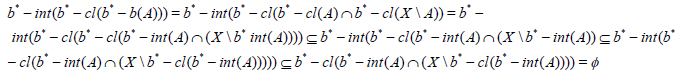Also if A∈b*C(X) Then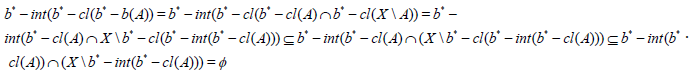Proposition 3.3: For a space (X,τ), A ⊆ X, then the sets A∩ b*-cl(X\A) and b*-cl(A)∩ (X\A) are b*- residual.

Proof: Since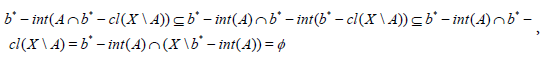Then A∩b*-cl(X\A) is residual. Similarly

b* − int(b* − cl(A)∩(X \ A)) ⊆ b* − int(b* − cl(A))∩b* − int(X \ A) = b* − cl(A)∩(X \ b* − cl(A)) =φ , and hence b*-c(A)∩(X\A) is b*- residual.

Theorem 3.11: The b*-boundary of any set contains the union of two b*-residual sets.

Proof: Let (X,τ) be a space and A ⊆ X. Then by Proposition (3.3), we have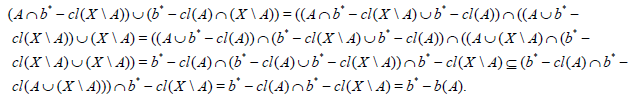#### References

Select your language of interest to view the total content in your interested language

### Article Usage

• Total views: 5031
• [From(publication date):
December-2016 - Nov 22, 2019]
• Breakdown by view type
• HTML page views : 4641Can't read the image? click here to refresh